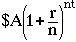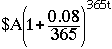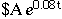Subject: figuring interest compounded daily How do I figure interest that is compounded daily? 8% compounded daily. Your prompt answer would be greatly appreciated. Debbie Bracken I am not a teacher or a student Hi Debbie, I assume that the interest rate is 8% per year, compounded daily. If you invest \$A at an interest rate of r for t years, compounded n times per year then the balance after the t years isHence if you invest \$A at 8% for t years compounded daily then the balance after t years would beA very good approximation to this is given by expressionwhere e, the base for the natural logarithms, is approximately 2.71828 Cheers, Penny Go to Math Central